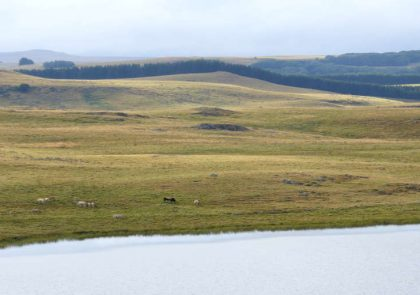# Blog

We provide practical tutorials on data mining, visualization and statistics for decision making.This R graphics tutorial shows how to customize a ggplot legend. you will learn how to: Change the legend title and text labels Modify the legend position. In the default setting of ggplot2, the legend is placed on the right of the plot. We’ll...

#### GGPlot Legend Title, Position and Labels

This R graphics tutorial shows how to customize a ggplot legend. you will learn how to: Change the legend title and text labels Modify the legend position. In the default setting of ggplot2, the legend is placed on the right of the plot. We’ll...This article describes how to format ggplot date axis using the R functions scale_x_date() and scale_y_date(). In this R graphics tutorial, you’ll learn how to: Change date axis labels using different combinations of days, weeks, months, year Modify date axis limits. Contents: Key ggplot2...

#### GGPlot Date Axis Customization

This article describes how to format ggplot date axis using the R functions scale_x_date() and scale_y_date(). In this R graphics tutorial, you’ll learn how to: Change date axis labels using different combinations of days, weeks, months, year Modify date axis limits. Contents: Key ggplot2...This article describes how to create a ggplot with a log scale. This can be done easily using the ggplot2 functions scale_x_continuous() and scale_y_continuous(), which make it possible to set log2 or log10 axis scale. An other possibility is the function scale_x_log10() and scale_y_log10(),...

#### GGPlot Log Scale Transformation

This article describes how to create a ggplot with a log scale. This can be done easily using the ggplot2 functions scale_x_continuous() and scale_y_continuous(), which make it possible to set log2 or log10 axis scale. An other possibility is the function scale_x_log10() and scale_y_log10(),...This article describes how to easily set ggplot axis ticks for both x and y axes. We’ll also explain how to rotate axis labels by specifying a rotation angle. In this R graphics tutorial, you will learn how to: Change the font style (size,...

#### GGPlot Axis Ticks: Set and Rotate Text Labels

This article describes how to easily set ggplot axis ticks for both x and y axes. We’ll also explain how to rotate axis labels by specifying a rotation angle. In this R graphics tutorial, you will learn how to: Change the font style (size,...This article describes R functions for changing ggplot axis limits (or scales). We’ll describe how to specify the minimum and the maximum values of axes. Among the different functions available in ggplot2 for setting the axis range, the coord_cartesian() function is the most preferred,...

#### GGPlot Axis Limits and Scales

This article describes R functions for changing ggplot axis limits (or scales). We’ll describe how to specify the minimum and the maximum values of axes. Among the different functions available in ggplot2 for setting the axis range, the coord_cartesian() function is the most preferred,...This article describes how to change ggplot axis labels (or axis title). This can be done easily using the R function labs() or the functions xlab() and ylab(). In this R graphics tutorial, you will learn how to: Remove the x and y axis...

#### GGPlot Axis Labels

This article describes how to change ggplot axis labels (or axis title). This can be done easily using the R function labs() or the functions xlab() and ylab(). In this R graphics tutorial, you will learn how to: Remove the x and y axis...This article describes how to add and change a main title, a subtitle and a caption to a graph generated using the ggplot2 R package. We’ll show also how to center the title position, as well as, how to change the title font size...

#### GGPlot Title, Subtitle and Caption

This article describes how to add and change a main title, a subtitle and a caption to a graph generated using the ggplot2 R package. We’ll show also how to center the title position, as well as, how to change the title font size...Cluster analysis is one of the important data mining methods for discovering knowledge in multidimensional data. The goal of clustering is to identify pattern or groups of similar objects within a data set of interest. Each group contains observations with similar profile according to...

#### Cluster Analysis in R: Practical Guide

Cluster analysis is one of the important data mining methods for discovering knowledge in multidimensional data. The goal of clustering is to identify pattern or groups of similar objects within a data set of interest. Each group contains observations with similar profile according to...Clustering methods are used to identify groups of similar objects in a multivariate data sets collected from fields such as marketing, bio-medical and geo-spatial. They are different types of clustering methods, including: Partitioning methods Hierarchical clustering Fuzzy clustering Density-based clustering Model-based clustering In...

#### Types of Clustering Methods: Overview and Quick Start R Code

Clustering methods are used to identify groups of similar objects in a multivariate data sets collected from fields such as marketing, bio-medical and geo-spatial. They are different types of clustering methods, including: Partitioning methods Hierarchical clustering Fuzzy clustering Density-based clustering Model-based clustering In...This article describes k-means clustering example and provide a step-by-step guide summarizing the different steps to follow for conducting a cluster analysis on a real data set using R software. We’ll use mainly two R packages: cluster: for cluster analyses and factoextra: for the...

#### Clustering Example: 4 Steps You Should Know

This article describes k-means clustering example and provide a step-by-step guide summarizing the different steps to follow for conducting a cluster analysis on a real data set using R software. We’ll use mainly two R packages: cluster: for cluster analyses and factoextra: for the...